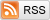# The Hottest Person in the Group

April 4, 2013

Suppose you think you're pretty good-looking. In fact, you think you're at about the 90th percentile--you're hotter than 90% of people and not as hot as the other 10%. If I put you with another nine random people, you'd think, at least heuristically, that you're probably the hottest one out of the ten. This is in fact not true.

The actual probability that you're the best-looking person in the group is the probability that none of the other nine people is in the top 10%, or $(1 - 1/10)^9 = 38.7\%$. So you'd actually be the hottest person in this group less than half the time. More generally, if only one out of every $n$ people is better-looking than you, then the probability that you're the best looking person in a group of $n$ people, including yourself, is $(1 - 1/n)^{n-1}$, which converges (quickly and monotonically!) to $1/e = 36.8\%$.

The converse problem is more flattering, though--suppose that whenever you find yourself in a random group with nine other people you actually are usually the best looking-person there. If we take the definition of "usually" to be 95% of the time, the de-facto scientific standard, then it's easy to calculate that you're actually at the 99.4th attractiveness percentile! (Since $0.95^{1/9} = 0.9943$).